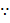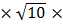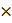# Two point charges q1 () and q2(−25are placed on the x-axis at x = 1 m and x = 4 m respectively. The electric field (in V/m) at a point y = 3 m on y-axis is,a. (−63) × 102 b. (81-81c. (63× 102 d. (−81×102

## Question ID - 50123 :- Two point charges q1 () and q2(−25are placed on the x-axis at x = 1 m and x = 4 m respectively. The electric field (in V/m) at a point y = 3 m on y-axis is,a. (−63) × 102 b. (81-81c. (63× 102 d. (−81×102

3537Let&are the vaues of electric field due to q1  and q2 Respectively magnitude of

E2 =E2=E2=9×103   V/m= 9×103 (− sinθ2tanθ2 = 3/4= 9×103Magnitude of==(9=9102=9102 [cos1()+sin1]

∴  tan=3

E1=9102E1=9102=102=1+2 =(63−27)102 V/m

Next Question :Book, Novel, Pen

(A) a       (B) b      (C) c       (D) d